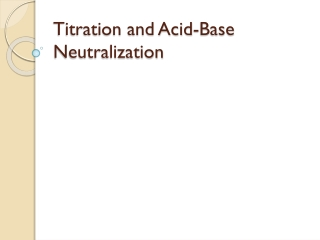DownloadDownload PresentationTitration and Acid-Base Neutralization

# Titration and Acid-Base Neutralization

Télécharger la présentation## Titration and Acid-Base Neutralization

- - - - - - - - - - - - - - - - - - - - - - - - - - - E N D - - - - - - - - - - - - - - - - - - - - - - - - - - -
##### Presentation Transcript

1. Titration and Acid-Base Neutralization

2. Acid Base Neutralization Reaction • Acid + Base  Water + Salt • Ex: HCl+ NaOH  H2O + NaCl

3. Example: Stomach antacids

4. Titration: • A laboratory method for determining the concentration of an unknown acid or base using a neutralisation reaction. • A standard solution,(a solution of known concentration), is used.

5. Equivalence Point • The point at which there are stoichiometrically equivalent amounts of acid and base. • [H+] = [OH-]

6. Buret Valve

7. Titration Acid with Phenolpthalein End-Point

8. Indicators • Indicators are chosen, such that they change colors at the range of the pH of interest. • For the NSW Titration competition, the only indicator used is phenolpthalein. • The solution itself at the end-point may be: • Basic, if the reaction involves a strong base and a weak acid. • Neutral, if the reaction involves a strong acid and a strong base. • Acidic, if the reaction involves a strong acid and a weak base.

9. Methods of Solving Titration Problems: a) using stoichiometry b) using the titration formula aMaVa=bMbVb.

10. Ex. 1 What is the concentration of HCl if 30.0 mL of 0.10 M NaOH neutralizes 50.0mL HCl? NaOH + HCl  H2O + NaCl Hint: Use aMaVa=bMbVb Ma= How many moles of HCl were used? Hint: #moles= MaVa , but convert the volume to L( 50mL=0.05L).

11. Ex. 2 A 20.0 mL solution of Sr(OH)2 is neutralized after 25.0 mL of standard 0.05 M HCl is added. What is the concentration of Sr(OH)2? 2 HCl + Sr(OH)2 2 H2O + SrCl2

12. Ex. 3 • How many mL of 0.20 M H3PO4 are needed to neutralize 55.0 mL of a 0.10 M solution of NaOH?

13. Ex. 4 • What volume of 0.20M Ca(OH)2 will neutralize 45.0 mL of a 1M solution of HClO3?